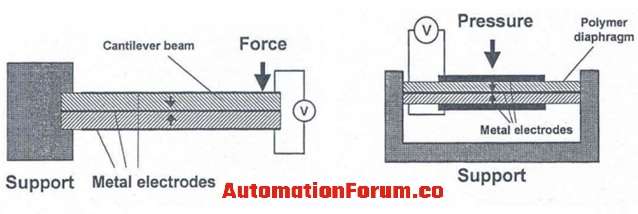# What is a piezoelectric sensor?A piezoelectric sensor can be used to measure the displacement, pressure, temperature, force, and strain. These sensors operate according to the piezoelectric effect, the piezoelectric effect is the formation of electric potential in a crystal if it is subjected to mechanical pressure. The crystals that are mostly used will be quartz, and barium titanate. The electric charge which is created will be proportional to the mechanical stress. The major function of a piezoelectric sensor is to convert the physical parameters such as acceleration or pressure into an electric signal. In some sensors, the physical parameters would directly act on the piezoelectric element while in the other device an acoustical signal establishes vibrations in the element and this vibration would be converted into an electric signal.

### What is a piezoelectric actuator and transducer?

In a piezoelectric actuator, the electric signal is converted into a physical displacement and this will be used to control the precision of the machine tool. The actuator can also be useful to control the hydraulic valves. In the case of a piezoelectric transducer, it would convert the electrical energy into vibrational mechanical energy and it could be vice versa too. The piezoelectric effect is reversible so the transducer can create ultrasonic sound from electrical energy and also it can convert the incoming sound into electrical energy. A piezoelectric transducer is used for atomizing liquids, medical diagnostics, etc.

### What is a piezoelectric pressure sensor?

The major part of a piezoelectric pressure sensor would be a diaphragm and a piezoelectric crystal. These sensors can detect the pressure by the displacement of a thin metal diaphragm. The diaphragm would cause a strain on the piezoelectric crystal when flexing due to the change in pressure. The electric charge that reaches the face of the crystal would be of opposite polarity and it would be proportional to the strain.

### What is a piezoelectric accelerometer?

This sensor uses a piezoelectric crystal and it would produce a voltage across the crystal if it is stressed. The piezoelectric crystal could be quartz, if there is an upward or downward movement in the housing of this device then the force would affect the piezoelectric element and by this, stress would act on the piezoelectric material and thus voltage is formed. This device is only capable to measure varying measurements and it can’t measure steady accelerations.

### What are the factors that must be considered while using a piezoelectric sensor?

Mostly these sensors are used to measure the dynamic pressure and they are not used for static pressure measurement. In order to do the proper operation of the sensor then the sensor alignment must be done properly. By doing the proper alignment of the sensor the sensor will be highly sensitive. We must not use this type of sensor for high-temperature applications. If we are using these sensors for high-temperature applications then we must either use recess mounting, baffled diaphragm, or thermal protection coating.

### How does a piezoelectric sensor works?

If a piezoelectric material is exposed to an electric field, then it could be lengthened or shortened according to the voltage. If we stretch or squeeze a piezoelectric crystal then the structure could be deformed and an electric charge will appear. So by using this property of the piezoelectric material we can measure displacement pressure etc. Mechanical stress would be applied to the crystal and the crystal would produce a signal proportional to the stress.

The micro-electric circuit will process the signal and this would be done either internally or externally. The signal condition would be done and this includes the conversion of the signal to a low impedance voltage, amplification, and filtering.

### What are the advantages of piezo-electric sensor?

• This sensor doesn’t need any external power source
• Wide amplitude range
• There are no moving parts so no wear
• Wide frequency range
• Highly sensitive
• It is compact
• Good linearity over the dynamic range
• Low output noise

### What are the disadvantages of piezo-electric sensor?

• This sensor is not capable to measure the static position
• Temperature variation in the crystal could affect the measurement
• Displacement is limited
• It needs high impedance amplification of the signal
• If it operates over long cables then it could affect the frequency response and it could create noise and distortion

### What are the applications of a piezo-electric sensor?

• It is used in accelerometers
• Sonar
• Ultrasonic actuator
• Traffic sensors
• Machine monitoring